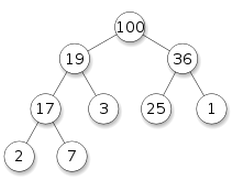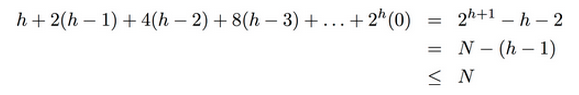# Priority Queue/Heap (优先队列/堆)小结

[TOC]

PQ又叫"堆"(heap), 但是可能优先队列这个名字更容易记忆它的用途: pq是一种队列, 不过不是先进先出(FIFO), 而是每次出队的元素永远是优先级最高的.

# logical structure of PQ

• 除了最后一层, 所有层都排满(没有非空节点)
• 最后一层的所有非空节点都排在左边# array representation of PQ

• `father(i) = i/2` 其中father(i)表示编号为i的节点的父节点的下标
• `leftchild(i) = i*2, rightchild(i) = i*2+1`

• 高度为lgN
• 第k层有 2^k 个节点 (root是第0层)
• 最后一层的节点对应的下标>=N/2

# PQ implementation

```public class PQ{// maxPQ of integers
int pq[];
int MAX_CAPACITY=1000;// if we use ArrayList we do not need MAX_CAPACITY
int size;// pq[size] is the index of last element (rightmost node in last level)
public PQ(){
pq = new int[MAX_CAPACITY+1];
size = 0;
}
public boolean isEmpty(){
return size==0;
}
public int top(){ // get top element
assert !isEmpty();
return pq;
}
public void add(int n); // insert element to PQ --> stay tuned
public int poll(); // get and remove top element --> stay tuned
}
```

### siftup

```private void siftup(){
int i = size;// i is the index of the newly added element
for(;i>1 && pq[i/2]<pq[i]; i/=2)
swap(pq, i/2, i);
}
```

### siftdown

siftdown的功能和siftup相反: 如果在最高处是一个优先级很低的元素, 需要将其"下放". 方法就是把它和子节点里面优先级较高的进行交换.

```private void siftdown(){
if(isEmpty()) return;// nothing to sift when empty
int i=1;// siftdown root node
while(i*2<=size){ // while the node is not in last level
int max=pq[i], j=i;// j is the element to swap
if (pq[i*2]>max) // left child
{j=i*2; max=pq[i*2];}
if (i*2+1<=size && pq[i*2+1]>max) // right node
{j=i*2+1; max=pq[i*2+1];}
if (j==i) return;// stop when node is bigger than both child
swap(pq, i, j);
i = j;
}
}
```

## implementation of add and poll

add

```public void add(int n){ // insert element to PQ
assert size+1<MAX_CAPACITY;
pq[++size] = n;
siftup();
}
```

poll

```public int poll(){ // get and remove top element
assert !isEmpty();
int top = pq;
pq = pq[size--];// put last element on the top
siftdown();
return top;
}
```

# PQ application

### heapify

```public static void heapify(int[] a){
int N = a.length;
for(int i=N/2;i<=1;i--){
siftdown(a, i);
}
}
```

→ 第k层节点有2^k个节点, 这一层的节点向下调整最多会进行h-k步, 所以计算量是一个求和表达式:
`Sigma( 2^k * (h-k) ) for k=0,...,h-1`(具体见 http://algs4.cs.princeton.edu/24pq/ 里面Q20的答案)

### top K elements of a stream

• if minPQ.size<K → `minPQ.add(n)`
• if minPQ.size==K → 比较n和minPQ.top():
• if n>minPQ.top(): `minPQ.poll(); minPQ.add(n)`
• else pass...

### median of a stream

⇒ 这样中位数或者是maxpq.top(), 或者是两个top的平均值了~

# more about heap

### more "flexible" heap

#### Part 6 of series «算法笔记»：

comments powered by Disqus